# Python - 面向对象编程 - MRO 方法搜索顺序

### 为什么会讲 MRO？

• 在讲多继承的时候：https://www.cnblogs.com/poloyy/p/15224912.html
• 有讲到， 当继承的多个父类拥有同名属性、方法，子类对象调用该属性、方法时会调用哪个父类的属性、方法呢？
• 这就取决于 Python 的 MRO 了

### 什么是 MRO

• MRO，method resolution order，方法搜索顺序
• 对于单继承来说，MRO 很简单，从当前类开始，逐个搜索它的父类有没有对应的属性、方法
• 所以 MRO 更多用在多继承时判断方法、属性的调用路径
• Python 中针对类提供了一个内置属性 __mro__ 可以查看方法搜索顺序

#### 实际代码

class A:
def test(self):
print("AAA-test")

class B:
def test(self):
print("BBB-test")

# 继承了三个类，B、A、还有默认继承的 object
class C(B, A):
...

# 通过类对象调用，不是实例对象！
print(C.__mro__)

# 输出结果
(<class '__main__.C'>, <class '__main__.B'>, <class '__main__.A'>, <class 'object'>)
1. 在搜索方法时，是按照 __mro__ 的输出结果从左往右的顺序查找的
2. 如果在当前类（Class C）中找到方法，就直接执行，不再搜索
3. 如果没有找到，就查找下一个类中（Class B）是否有对应的方法，如果找到，就直接执行，不再搜素
4. 如果找到最后一个类（Class object）都没有找到方法，程序报错

#### 类图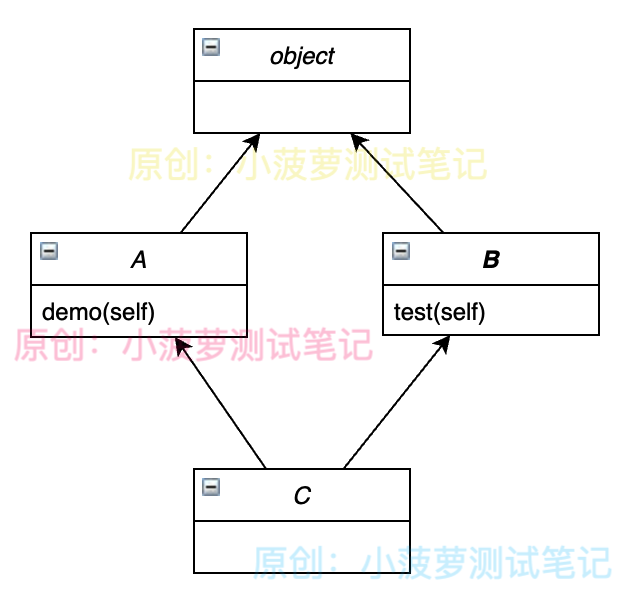### MRO 算法

Python 发展到现在经历了三种算法

1. 旧式类 MRO 算法：从左往右，采用深度优先搜索（DFS），从左往右的算法，称为旧式类的 MRO
2. 新式类 MRO 算法：自 Python 2.2 版本开始，新式类在采用深度优先搜索算法的基础上，对其做了优化
3. C3 算法：自 Python 2.3 版本，对新式类采用了 C3 算法；由于 Python 3.x 仅支持新式类，所以该版本只使用 C3 算法

#### 什么是旧式类，新式类

https://www.cnblogs.com/poloyy/p/15226425.html

### 旧式类 MRO 算法

#### 实际代码

# 旧式类算法
class A:
def test(self):
print("CommonA")

class B(A):
pass

class C(A):
def test(self):
print("CommonC")

class D(B, C):
pass

D().test()

# python2 下的运行结果
CommonA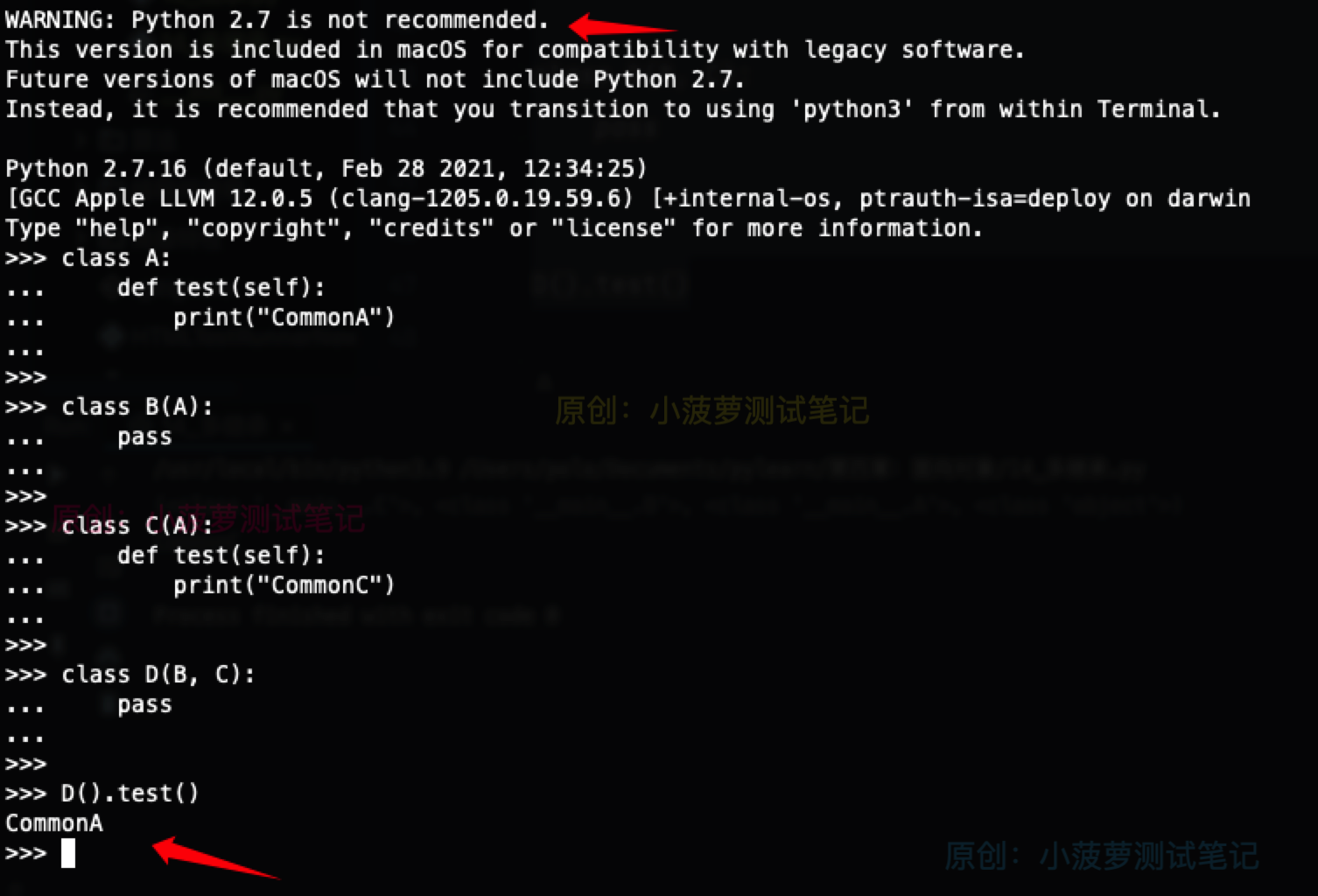#### 类图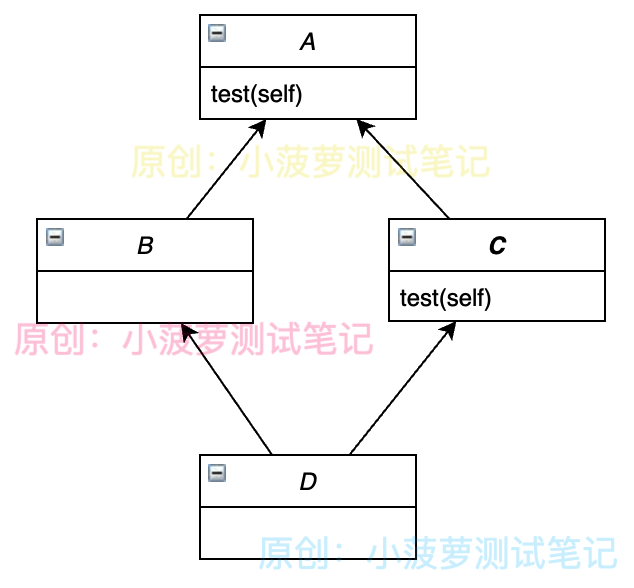#### 分析

• 通过类图可以看到，此程序中的 4 个类是一个“菱形”继承的关系
• 当使用 D 类实例对象访问 test() 方法时，根据深度优先算法，搜索顺序为  D->B->A->C->A
• 因此，旧式类 MRO 算法最先搜索得到 test() 方法是在 A 类里面，所以最终输出结果为 CommonA

### 新式类 MRO 算法

• 为解决旧式类 MRO 算法存在的问题，Python 2.2 版本推出了新的计算新式类 MRO 的方法
• 它仍然采用从左至右的深度优先遍历，但是如果遍历中出现重复的类，只保留最后一个

#### 以上面的代码栗子来讲

• 深度优先遍历，搜索顺序为 D->B->A->C->A
• 因为顺序中有 2 个 A，因此只保留最后一个
• 最终搜索顺序为 D->B->C->A

#### 实际代码

class X(object):
pass

class Y(object):
pass

class A(X, Y):
pass

class B(Y, X):
pass

class C(A, B):
pass
• 深度优先遍历后的搜索顺序为： C->A->X->object->Y->object->B->Y->object->X->object
• 相同取后者的搜索顺序为： C->A->B->Y->X->object

• A： A->X->Y->object
• B： B->Y->X->object
• C： C->A->B->X->Y->object

#### 在 python2 中运行这段代码的报错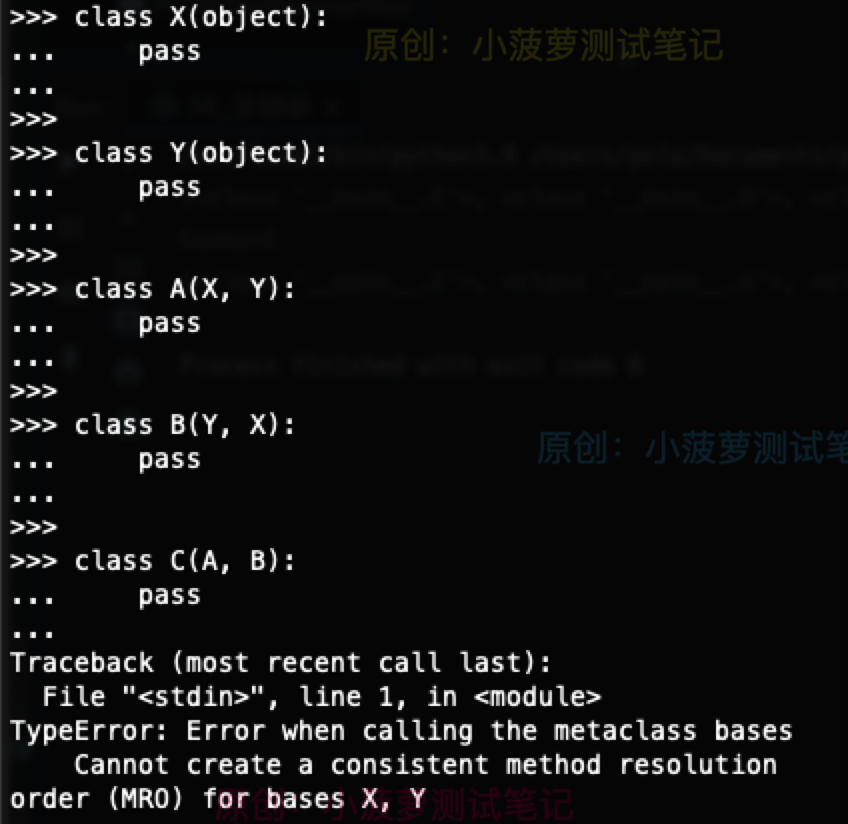#### 在 python3 中运行这段代码的报错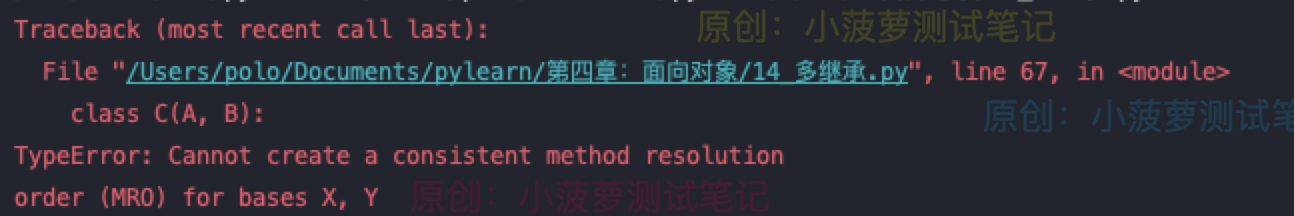### C3 MRO 算法

• 为解决前面两个算法的问题，Python 2.3 采用了 C3 方法来确定方法搜索顺序
• 多数情况下，如果别人提到 Python 中的 MRO，指的都是 C3 算法

#### 将上面第一个栗子的代码放到 python3 中运行

class A:
def test(self):
print("CommonA")

class B(A):
pass

class C(A):
def test(self):
print("CommonC")

class D(B, C):
pass

D().test()

# 输出结果
CommonC

#### 简单了解下 C3 算法

• A：L[A] = merge(A , object)
• B：L[B] = B + merge(L[A] , A)
• C：L[C] = C + merge(L[A] , A)
• D：L[D] = D + merge(L[B] , L[C] , B , C)

#### merge 的运算方式

1. 将 merge 第一个列表的头元素（如 L[A] 的头），记作 H
2. 如果 H 出现在 merge 其他列表的头部，则将其输出，并将其从所有列表中删除
3. 如果 H 只出现一次，那么也将其输出，并将其从所有列表中删除
4. 如果 H 出现在 merge 其他列表的非头部，则取下一个列表的头元素记作 H，然后回到步骤二
5. 最后回到步骤一，重复以上步骤

### 简单类 MRO 的计算栗子

class B(object): pass

print(B.__mro__)

(<class '__main__.B'>, <class 'object'>)

#### MRO 计算方式

L[B] = L[B(object)]
= B + merge(L[object])
= B + L[object]
= B object

### 单继承 MRO 的计算栗子

# 计算 MRO
class B(object): pass

class C(B): pass

print(C.__mro__)

(<class '__main__.C'>, <class '__main__.B'>, <class 'object'>)

#### MRO 计算方式

L[C] = C + merge(L[B])
= C + L[B]
= C B object

### 多继承 MRO 的计算栗子

O = object

class F(O): pass

class E(O): pass

class D(O): pass

class C(D, F): pass

class B(D, E): pass

class A(B, C): pass

print(C.__mro__)
print(B.__mro__)
print(A.__mro__)

# 输出结果
(<class '__main__.C'>, <class '__main__.D'>, <class '__main__.F'>, <class 'object'>)
(<class '__main__.B'>, <class '__main__.D'>, <class '__main__.E'>, <class 'object'>)
(<class '__main__.A'>, <class '__main__.B'>, <class '__main__.C'>, <class '__main__.D'>, <class '__main__.E'>, <class '__main__.F'>, <class 'object'>)

#### O 类、object 类 MRO 计算

L[O] = O = object

#### D、E、F 类 MRO 计算

L[D] = D + merge(L[O])
= D O

#### C 类 MRO 计算

L[C] = L[C(D, F)]
= C + merge(L[D], L[F], DF)
# 从前面可知 L[D] 和 L[F] 的结果
= C +  merge(DO, FO, DF)
# 因为 D 是顺序第一个并且在几个包含 D 的 list 中是 head，
# 所以这一次取 D 同时从列表中删除 D
= C + D + merge(O, FO, F)
# 因为 O 虽然是顺序第一个但在其他 list (FO)中是在尾部, 跳过
# 改为检查第二个list FO
# F 是第二个 list 和其他 list 的 head
# 取 F 同时从列表中删除 F
= C + D + F + merge(O)
= C D F O

#### B 类 MRO 计算

L[B] = L[B(D, E)]
= B + merge(L[D], L[E], DE)
= B + merge(DO, EO, DE)
= B + D + merge(O, EO, E)
= B + D + E + merge(O)
= B D E O

#### A 类 MRO 计算

L[A] = L[A(B,C)]
= A + merge(L[B], L[C], BC)
= A + merge( BDEO, CDFO, BC )
= A + B + merge( DEO, CDFO, C )
# D 在其他列表 CDFO 不是 head，所以跳过到下一个列表的 头元素 C
= A + B + C + merge( DEO, DFO )
= A + B + C + D + merge( EO, FO )
= A + B + C + D + E + merge( O, FO )
= A + B + C + D + E + F + merge( O )
= A B C D E F O

### 多继承 MRO 的计算栗子二

O = object

class F(O): pass

class E(O): pass

class D(O): pass

class C(D, F): pass

class B(E, D): pass

class A(B, C): pass

print(C.__mro__)
print(B.__mro__)
print(A.__mro__)

# 输出结果
(<class '__main__.C'>, <class '__main__.D'>, <class '__main__.F'>, <class 'object'>)
(<class '__main__.B'>, <class '__main__.E'>, <class '__main__.D'>, <class 'object'>)
(<class '__main__.A'>, <class '__main__.B'>, <class '__main__.E'>, <class '__main__.C'>, <class '__main__.D'>, <class '__main__.F'>, <class 'object'>)

#### O 类、object 类 MRO 计算

L[O] = O = object

#### D、E、F 类 MRO 计算

L[D] = D + merge(L[O])
= D O

#### C 类 MRO 计算

L[C] = L[C(D, F)]
= C + merge(L[D], L[F], DF)
= C + merge(DO, FO, DF)
= C + D + merge(O, FO, F)
= C + D + F + merge(O)
= C D F O

#### B 类 MRO 计算

L[B] = L[B(E, D)]
= B + merge(L[E], L[D], ED)
= B + merge(EO, DO, ED)
= B + E + merge(O, DO, D)
= B + E + D + merge(O)
= B E D O

#### A 类 MRO 计算

L[A]  = L[A(B, C)]
= A + merge(L[B], L[C], BC)
= A + merge(BEDO, CDFO, BC)
= A + B + merge(EDO, CDFO, C)
= A + B + E + merge(DO,CDFO, C)
= A + B + E + C + merge(O,DFO)
= A + B + E + C + D + merge(O, FO)
= A + B + E + C + D + F + merge(O)
= A B E C D F O

posted @ 2021-09-06 22:41  小菠萝测试笔记  阅读(254)  评论(0编辑  收藏  举报